# 8 Some solutions to the consistency equations

Equations (130)-(135) and (141)-(142), required by the consistency of the first-order deformation, possess the following classes of solutions, interesting from the point of view of cross-couplings between the BF field sector and the tensor field with the mixed symmetry.

I.
The real constants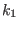and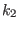are arbitrary (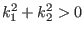), functions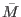andare some arbitrary, real, smooth functions of the undifferentiated scalar field, and(208)(209)

The above formulas allow one to infer directly the solution in the general case. This class of solutions can be equivalently reformulated as: the real constantsandare arbitrary (), functionsand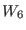are some arbitrary, real, smooth functions of the undifferentiated scalar field, and(210)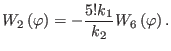(211)

The last formulas are useful at writing down the solution in the particular case.

II.
The real constantsandare arbitrary (), functionsandare some arbitrary, real, smooth functions of the undifferentiated scalar field, and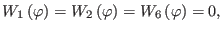(212)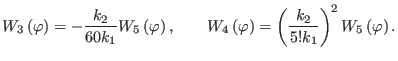(213)

The above formulas allow one to infer directly the solution in the general case. This class of solutions can be equivalently reformulated as: the real constantsandare arbitrary (), functionsand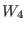are some arbitrary, real, smooth functions of the undifferentiated scalar field, and(214)(215)

The last formulas are useful at writing down the solution in the particular case.

III.
The real constantsandare arbitrary (), functionsandare some arbitrary, real, smooth functions of the undifferentiated scalar field, and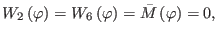(216)(217)

The above formulas allow one to infer directly the solution in the general case. This class of solutions can be equivalently reformulated as: the real constantsandare arbitrary (), functionsandare some arbitrary, real, smooth functions of the undifferentiated scalar field, and(218)(219)

The last formulas are useful at writing down the solution in the particular case.

For all classes of solutions the emerging interacting theories display the following common features:

1.
there appear nontrivial cross-couplings between the BF fields and the tensor field with the mixed symmetry;

2.
the gauge transformations are modified with respect to those of the free theory and the gauge algebras become open (only close on-shell);

3.
the first-order reducibility functions are changed during the deformation process and the first-order reducibility relations take place on-shell.

Nevertheless, there appear the following differences between the above classes of solutions at the level of the higher-order reducibility:

a)
for class I the second-order reducibility functions are modified with respect to the free ones and the corresponding reducibility relations take place on-shell. The third-order reducibility functions remain those from the free case and hence the associated reducibility relations hold off-shell;

b)
for class II both the second- and third-order reducibility functions remain those from the free case and hence the associated reducibility relations hold off-shell;

c)
for class III all the second- and third-order reducibility functions are deformed and the corresponding reducibility relations only close on-shell.

Ashkbiz Danehkar
2018-03-26